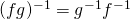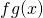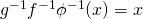# Finding the inverse for a composite functionMany students know how to find the inverse of a regular function. We simply letthen proceed to makethe subject. Some might not know that if you want to find the inverse of the composite function, knowing thatwill be useful. Do note the switch in position of the functions.

At times, it might be really impossible to make out the inverse ofby doing the regularand then proceeding to makethe subject. Thus, this formula will come in handy.

The following is a simple proof. Assuming all the following composite functions exists.

LetThenWe have thatConsideringNot readable? Change text.